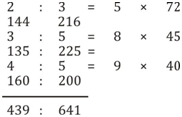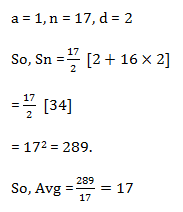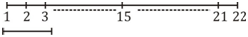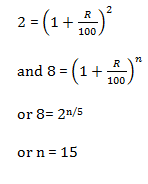# SSC Quantitative Aptitude Questions (Day-65)

Dear Aspirants, you can find the Quantitative Aptitude questions with detailed explanations for the SSC exams. Nowadays the competitive level of the exam has been increasing consistently. Due to the great demand for the government job, the level of the toughness reached greater. Candidates have to enhance the preparation process in order to drive in the right path. It doesn’t need to clear the prescribed cutoff. You must have to score good marks more than the cut off marks to get into the final provisional list. Here we have updating the Quantitative Aptitude questions with detailed explanations on a daily basis. You can practice with us and measure your level of preparation. According to that you can sculpt yourself in a proper way. SSC aspirants kindly make use of it and grab your success in your career.

Start Quiz

1) Three bottles of equal capacity contain mixture of milk and water in ratio 2 : 3, 3 : 5 and 4 : 5 respectively. These three bottles are emptied into a largebottle. What is the ratio of milk and water respectively in the large bottle?

(a) 439 : 1080

(b) 439 : 641

(c) 439 : 360

(d) 439 : 79

2) What is the average of ﬁrst 17 odd numbers?

(a) 16

(b) 17

(c) 18

(d) 19

3) The average of 4 consecutive even numbers is 51. What is the third number?

(a) 46

(b) 52

(c) 54

(d) 56

4) Rohit buys an old bat for Rs 1230 and spends Rs 180 on its repairs. If he sells it for Rs 1128, then what is his loss percentage?

(a) 12.5

(b) 15

(c) 20

(d) 25

5) A trader sells two articles for Rs 1875 each. If he gains 25% on one and losses 25% on other, then what is the value (in Rs) of the loss?

(a) 150

(b) 250

(c) 400

(d) 500

6) If the price of milk increases from Rs 60/kg to Rs 70/kg, then by what percentage a person should decrease the consumption of milk so that expenditureremains same?

(a) 16.66

(b) 14.28

(c) 12.33

(d) 17.33

7) If length of a rectangle is increased by 20% and breadth is increased by 30%, then what will be the percentage increase in the area of rectangle?

(a) 50

(b) 46

(c) 56

(d) 62

8) Two cars are travelling towards each other with speeds of 45 km/hr and 63 km/hr respectively. What is the distance (in kilometres) between the two carsten second before they collide?

(a) 0.5

(b) 0.2

(c) 0.3

(d) 0.45

9) 29 boys are standing in a line such that distance between any two consecutive boys is same. A teacher takes 7 seconds to reach from ﬁrst boy to 15thboy. How much more time (in seconds) will he take to reach the 22nd boy?

(a) 2.5

(b) 3

(c) 3.5

(d) 4

10) If a certain sum becomes 2 times in 5 years at compound interest, then in how many years, it will become 8 times?

(a) 10

(b) 20

(c) 15

(d) 30a – 3, a – 1, a + 1, a+3

4a/4 = 51 ⟹ a = 51

51 + 1 = 52

CP = 1230 + 180 = 1410

SP = 1128

Loss = 282

So,

% L = 282/1410 × 100

loss = 20%

clearly,

1.25 C1 = 1875

And,

0.75C2 = 1875

⟹ C1 = 1500 and C2 = 2500

So, C1 + C2 = 4000

And S1 + S2 = 3750

Loss = 250

Expenditure = Cost × Consumption

Exp = 60 × x

Exp = 70 × (x – a)

60x = 70(x – a)

Or 70a = 10x

Or a/x = 1/7

∴ a/x  % = 1/7 × 100 = 14.28%

% Increase = 20 + 30 + 6= 56%

distance travelled by them in 10 sec

= (45 + 63) × 10/3600

= 108/360 = 12/40 = 0.3 km

Note: This is the sum of distance travelled by them in last 10 secondsLet distance b/wn  each boy be 1m.

So, 14m travelled in = 7 sec

So, 21m travelled in = 7/14 × 21 = 10.5 sec

∴ 3.5 sec more required.